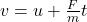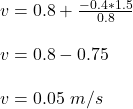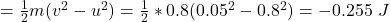## A fancart of mass 0.8 kg initially has a velocity of < 0.8, 0, 0 > m/s. Then the fan is turned on, and the air exerts a constant force

Question

A fancart of mass 0.8 kg initially has a velocity of < 0.8, 0, 0 > m/s. Then the fan is turned on, and the air exerts a constant force of < −0.4, 0, 0 > N on the cart for 1.5 seconds. What is the change in kinetic energy of the fancart over this 1.5 second interval?

in progress 0
5 months 2021-08-13T17:35:04+00:00 1 Answers 46 views 0

The change in kinetic energy of the fancart over 1.5 second time interval is -0.255 J

Explanation:

Given;

mass of fancart, m = 0.8 kg

initial velocity of fancart, u = < 0.8, 0, 0 > m/s

force exerted by air on fancart, F = < −0.4, 0, 0 > N

duration of the force, t =  1.5 seconds

The change in kinetic energy of the fancart over 1.5 second time interval;

Determine the final velocity, v

F = ma

a = F/m

Apply equation of motion;

v = u + atChange in Kinetic energy = final kinetic energy – initial kinetic energy

ΔKE = KE (final) – KE(initial)

ΔKETherefore, the change in kinetic energy of the fancart over 1.5 second time interval is -0.255 J